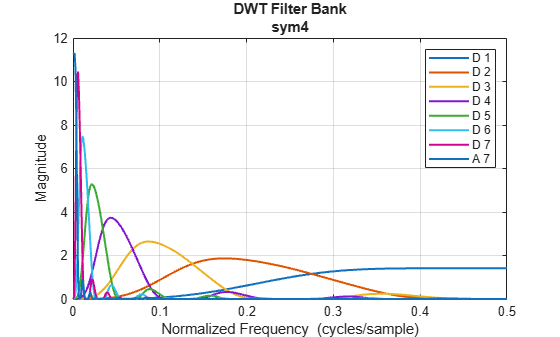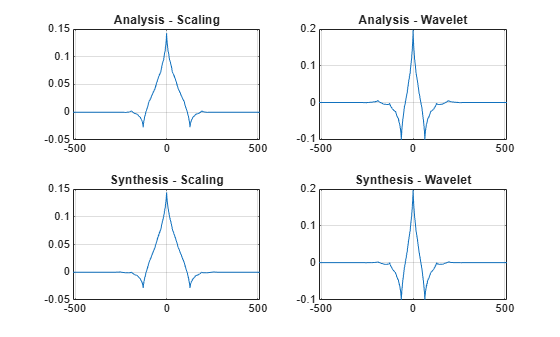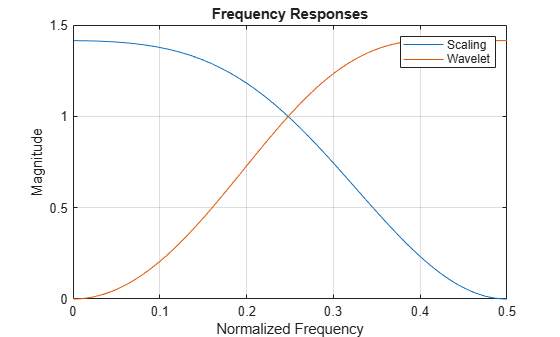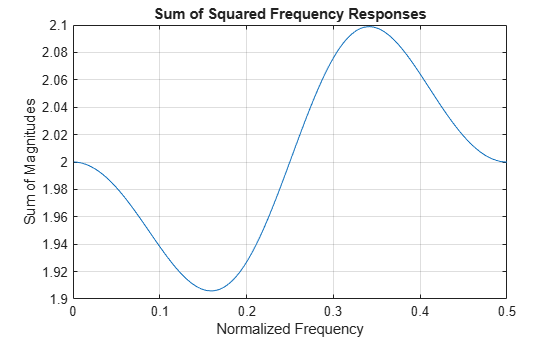# dwtfilterbank

Discrete wavelet transform filter bank

## Description

Use `dwtfilterbank` to create a discrete wavelet transform (DWT) filter bank

• Visualize wavelets and scaling functions in time and frequency.

• Measure the 3-dB bandwidths of the wavelet and scaling functions. You can also measure energy concentration of the wavelet and scaling functions in the theoretical DWT passbands.

• Create a DWT filter bank using your own custom filters. You can determine whether the filter bank is orthogonal or biorthogonal.

• Determine the frame bounds of the filter bank.

## Creation

### Syntax

``fb = dwtfilterbank``
``fb = dwtfilterbank(Name,Value)``

### Description

example

````fb = dwtfilterbank` create a discrete wavelet transform (DWT) filter bank. The default filter bank is designed for a signal with 1024 samples. The default filter bank uses the analysis (decomposition) sym4 wavelet and scaling filter with seven resolution levels.```

example

````fb = dwtfilterbank(Name,Value)` creates a DWT filter bank `fb` with properties specified by one or more `Name,Value` pair arguments. Properties can be specified in any order as `Name1,Value1,...,NameN,ValueN`. Enclose each property name in quotes.For example, ```fb = dwtfilterbank('SignalLength',1000,'Wavelet','bior4.4')``` creates a DWT filter bank for signals of length 1000 using the biorthogonal `bior4.4` wavelet. NoteYou cannot change a property value of an existing filter bank. For example, if you have a filter bank `fb` for the `sym4` wavelet, you must create a second filter bank `fb2` for the `coif5` wavelet. You cannot assign a different `Wavelet` to `fb`. ```

## Properties

expand all

Signal length, specified as a positive integer greater than or equal to 2.

Example: `'SignalLength',768`

Data Types: `double`

Name of wavelet used to construct the filter bank, specified as a character vector or string scalar. `Wavelet` is an orthogonal or biorthogonal wavelet recognized by `wavemngr` or `'Custom'`.

To use a wavelet filter not recognized by `wavemngr`, set the `Wavelet` property to `'Custom'` and specify the CustomWaveletFilter and CustomScalingFilter properties.

Example: `'Wavelet','bior4.4'`

Data Types: `char` | `string`

Wavelet filter type, specified as one of `'Analysis'` or `'Synthesis'`. `'Analysis'` uses the decomposition filters returned by `wfilters`. `'Synthesis'` uses the reconstruction filters.

Wavelet transform level, specified as a positive integer less than or equal to `floor(log2(SignalLength))`. For a signal of length 1024 and the `sym4` wavelet, the default level is 7.

By default the level is equal to `floor(log2(SignalLength/(L-1)))` where L is the length of the wavelet filter associated with `Wavelet`. For wavelets recognized by `wavemngr`, the transform level is equal to `wmaxlev(SignalLength,Wavelet)`. If `floor(log2(SignalLength/(L-1)))` is less than or equal to 0, `Level` defaults to `floor(log2(SignalLength))`.

Sampling frequency in hertz, specified as a positive scalar. If unspecified, frequencies are in cycles/sample and the Nyquist frequency is ½.

Example: `'SamplingFrequency',5`

Data Types: `double`

Custom wavelet filter coefficients, specified as a real-valued column vector or matrix. `CustomWaveletFilter` must be an even-length column vector for an orthogonal wavelet or a two-column matrix with an even number of rows for a biorthogonal wavelet.

This property applies only when `Wavelet` is set to `'Custom'`.

Custom scaling filter coefficients, specified as a real-valued column vector or matrix. `CustomScalingFilter` must be an even-length column vector for an orthogonal wavelet or a two-column matrix with an even number of rows for a biorthogonal wavelet.

This property applies only when `Wavelet` is set to `'Custom'`.

## Object Functions

 `dwtpassbands` DWT filter bank passbands `filters` DWT filter bank filters `framebounds` DWT filter bank frame bounds `freqz` DWT filter bank frequency responses `isBiorthogonal` Determine if DWT filter bank is biorthogonal `isOrthogonal` Determine if DWT filter bank is orthogonal `powerbw` DWT filter bank power bandwidth `qfactor` DWT filter bank quality factor `scalingfunctions` DWT filter bank time-domain scaling functions `wavelets` DWT filter bank time-domain wavelets `waveletsupport` DWT filter bank time supports

## Examples

collapse all

Create a DWT filter bank using default values.

`fb = dwtfilterbank`
```fb = dwtfilterbank with properties: Wavelet: 'sym4' SignalLength: 1024 Level: 7 SamplingFrequency: 1 FilterType: 'Analysis' CustomWaveletFilter: [] CustomScalingFilter: [] ```

Plot the magnitude frequency responses of the wavelets and coarsest-scale scaling function. Open the plot in a separate figure window. The plot legend in the window is interactive. To hide a particular frequency response, click on its name.

`freqz(fb)`

Obtain and plot the time-centered wavelets corresponding to the wavelet bandpass filters.

```[psi,t] = wavelets(fb); plot(t,psi') grid on title('Time-Centered Wavelets') xlabel('Time') ylabel('Magnitude')```This example shows how to create a DWT filter bank using custom biorthogonal wavelet filters.

Two pairs of analysis (decomposition) and synthesis (reconstruction) filters are associated with a biorthogonal wavelet. Each pair consists of a lowpass and highpass filter. Specify the analysis and synthesis filters for the nearly-orthogonal biorthogonal wavelets based on the Laplacian pyramid scheme of Burt and Adelson (Table 8.4 on page 283 in ). Because the toolbox requires that all filters associated with a biorthogonal wavelet or an orthogonal wavelet have the same even length, the filters are prepended and appended with 0s.

```Hd = [0 -1 5 12 5 -1 0 0]/20*sqrt(2); Gd = [0 3 -15 -73 170 -73 -15 3]/280*sqrt(2); Hr = [0 -3 -15 73 170 73 -15 -3]/280*sqrt(2); Gr = [0 -1 -5 12 -5 -1 0 0]/20*sqrt(2);```

`Hd` and `Gd` are the lowpass and highpass decomposition filters, respectively. `Hr` and `Gr` are the lowpass and highpass reconstruction filters, respectively.

Construct analysis and synthesis DWT filter banks using the biorthogonal filters. Confirm the filter banks are biorthogonal and not orthogonal.

```fbAna = dwtfilterbank('Wavelet','Custom',... 'CustomScalingFilter',[Hd' Hr'],'CustomWaveletFilter',[Gd' Gr']); fbSyn = dwtfilterbank('Wavelet','Custom',... 'CustomScalingFilter',[Hd' Hr'],'CustomWaveletFilter',[Gd' Gr'],... 'FilterType','Synthesis'); fprintf('fbAna: isOrthogonal = %d\tisBiorthogonal = %d\n',... isOrthogonal(fbAna),isBiorthogonal(fbAna));```
```fbAna: isOrthogonal = 0 isBiorthogonal = 1 ```
```fprintf('fbSyn: isOrthogonal = %d\tisBiorthogonal = %d\n',... isOrthogonal(fbSyn),isBiorthogonal(fbSyn ));```
```fbSyn: isOrthogonal = 0 isBiorthogonal = 1 ```

Obtain the wavelet and scaling functions of both filter banks. Plot the wavelet and scaling functions at the coarsest scales.

```[fbAna_phi,t] = scalingfunctions(fbAna); [fbAna_psi,~] = wavelets(fbAna); [fbSyn_phi,~] = scalingfunctions(fbSyn); [fbSyn_psi,~] = wavelets(fbSyn); subplot(2,2,1) plot(t,fbAna_phi(end,:)) grid on title('Analysis - Scaling') subplot(2,2,2) plot(t,fbAna_psi(end,:)) grid on title('Analysis - Wavelet') subplot(2,2,3) plot(t,fbSyn_phi(end,:)) grid on title('Synthesis - Scaling') subplot(2,2,4) plot(t,fbSyn_psi(end,:)) grid on title('Synthesis - Wavelet')```Compute the framebounds of the two filter banks. Since the filters are associated with biorthogonal wavelets, the framebounds will not equal 1.

`[a1,a2] = framebounds(fbAna)`
```a1 = 0.9505 ```
```a2 = 1.0211 ```
`[b1,b2] = framebounds(fbSyn)`
```b1 = 0.9800 ```
```b2 = 1.0528 ```

Obtain the frequency responses of the scaling and wavelets filters in the analysis filter bank. Plot up to Nyquist the magnitude frequency responses of the scaling and wavelet filters at the finest scale.

```[psidft,f,phidft] = freqz(fbAna); flen = length(f); figure plot(f(flen/2+1:end),abs(phidft(1,flen/2+1:end))) hold on plot(f(flen/2+1:end),abs(psidft(1,flen/2+1:end))) grid on legend('Scaling','Wavelet') title('Frequency Responses') xlabel('Normalized Frequency') ylabel('Magnitude')```Zoom in and confirm the magnitude frequency responses at the point of intersection are not magnitude equal to 1. Plot the sum of the squared magnitudes of the frequency responses. Because the scaling (lowpass) and wavelet (highpass) filters do not form an orthogonal quadrature mirror filter pair, the sum does not equal to 2 at all frequencies.

```figure plot(f(flen/2+1:end),abs(phidft(1,flen/2+1:end)).^2 + abs(psidft(1,flen/2+1:end)).^2) grid on title('Sum of Squared Frequency Responses') xlabel('Normalized Frequency') ylabel('Sum of Magnitudes')```Daubechies, I. Ten Lectures on Wavelets. CBMS-NSF Regional Conference Series in Applied Mathematics. Philadelphia, PA: Society for Industrial and Applied Mathematics, 1992.